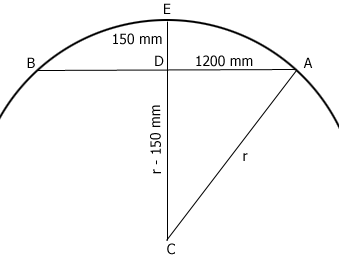SEARCH HOMEMath Central Quandaries & QueriesQuestion from Tim: Can I find the radius of a circle, if I have a chord of 2400mm, and a depth between the center of the chord and the perimeter of the circle of 150mm ? Thanks TimHi Tim,

In the diagram below triangle ADC is a right triangle and the length of CD is (r - 150) mm.Pythagoras theorem gives you

$1200^2 + (r - 150)^2 = r^2$

Expanding the left side gives

$1200^2 + r^2 - 300 r + 150^2 = r^2$

Simplifying gives

$300 r = 1200^2 + 150^2 = 1462500$

and hence

$r = \frac{1462500}{300} = 4875 \mbox{ mm}$

PennyMath Central is supported by the University of Regina and The Pacific Institute for the Mathematical Sciences.